# Primitive root modulo n facts for kids

Kids Encyclopedia Facts

In modular arithmetic, a number g is a primitive root modulo n, if every number m from 1..(n-1) can be expressed in the form of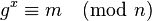$g^x\equiv m \pmod n$. As an example, 3 is a primitive root modulo 7: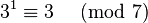$3^1 \equiv 3\ \pmod 7$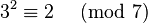$3^2 \equiv 2\ \pmod 7$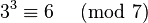$3^3 \equiv 6\ \pmod 7$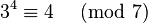$3^4 \equiv 4\ \pmod 7$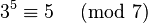$3^5 \equiv 5\ \pmod 7$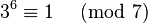$3^6 \equiv 1\ \pmod 7$

All the elements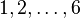$1, 2, \ldots, 6$ of the group modulo 7 can be expressed that way. The number 2 is no primitive root modulo 7, because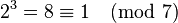$2^3=8 \equiv 1 \pmod 7$

and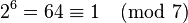$2^6=64 \equiv 1 \pmod 7$Primitive root modulo n Facts for Kids. Kiddle Encyclopedia.